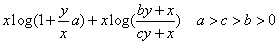# How to express this formula by CVX？`xlog(1+ ay/x)` can be expressed

` -rel_entr(x,x+ay)`

`xlog((by+x)/(cy+x))` can be expressed

` 1/(1-a/c)*(rel_entr(a*y+x,c*y+x)+a/c* rel_entr(c*y+x,a*y+x)`

therefore, the problem can be expressed

` 1/(1-a/c)*(rel_entr(a*y+x,c*y+x)+a/c* rel_entr(c*y+x,a*y+x) - rel_entr(x,x+ay)` ?

“Correct” or not, you’re not going to be able to subtract two nonnegative rel_entr terms. That is `{convex expression} - {convex expression}`, which is not allowed by CVX’s DCP rules.

The first term of the original expression is concave. The 2nd term is not concave; perhaps it is convex. The overall expression is not convex; perhaps it is concave. Therefore, if it is concave, you will have to formulate a single CVX expression for the overall expression,

But your first step is to prove this expression is concave (as opposed to neither concave nor convex).

Then, what method should I use to solve this problem?

If you want to solve a non-convex optimization problem, use a non-convex local or global optimization solver, for instance under YALMIP.